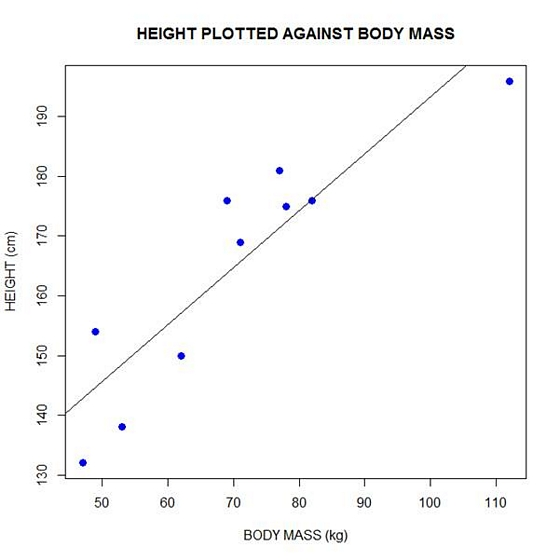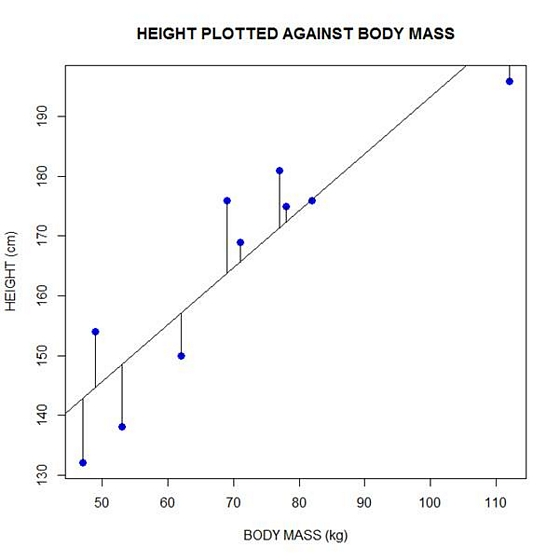# R Is Not So Hard! A Tutorial, Part 3: Regressions and PlotsIn Part 2 of this series, we created two variables and used the lm() command to perform a least squares regression on them, treating one of them as the dependent variable and the other as the independent variable. Here they are again.

`height = c(176, 154, 138, 196, 132, 176, 181, 169, 150, 175)`
`bodymass = c(82, 49, 53, 112, 47, 69, 77, 71, 62, 78)`

Today we learn how to obtain useful diagnostic information about a regression model and then how to draw residuals on a plot. As before, we perform the regression.

`lm(height ~ bodymass)`
Call:
lm(formula = height ~ bodymass)

Coefficients:
(Intercept) bodymass
98.0054 0.9528
Now let’s find out more about the regression. First, let’s store the regression model as an object called mod and then use the summary() command to learn about the regression.

`mod <- lm(height ~ bodymass)`

`summary(mod)`

Here is what R gives you.

Call:
lm(formula = height ~ bodymass)
Residuals:
Min 1Q Median 3Q Max
-10.786 -8.307 1.272 7.818 12.253

Coefficients:
Estimate Std. Error t value Pr(>|t|)
(Intercept) 98.0054 11.7053 8.373 3.14e-05 ***
bodymass 0.9528 0.1618 5.889 0.000366 ***

Signif. codes: 0 ‘***’ 0.001 ‘**’ 0.01 ‘*’ 0.05 ‘.’ 0.1 ‘ ’ 1

Residual standard error: 9.358 on 8 degrees of freedom
Multiple R-squared: 0.8126, Adjusted R-squared: 0.7891
F-statistic: 34.68 on 1 and 8 DF, p-value: 0.0003662
R has given you a great deal of diagnostic information about the regression. The most useful of this information are the coefficients themselves, the Adjusted R-squared, the F-statistic and the p-value for the model.

Now let’s use R’s predict() command to create a vector of fitted values.

`regmodel <- predict(lm(height ~ bodymass))`

`regmodel`

Here are the fitted values:

1 2 3 4 5 6 7 8 9 10
176.1334 144.6916 148.5027 204.7167 142.7861 163.7472 171.3695 165.6528 157.0778 172.3222

Now let’s plot the data and regression line again.

`plot(bodymass, height, pch = 16, cex = 1.3, col = "blue", main = "HEIGHT PLOTTED AGAINST BODY MASS", xlab = "BODY MASS (kg)", ylab = "HEIGHT (cm)")`

`abline(lm(height ~ bodymass))`We can plot the residuals using R’s for loop and a subscript k that runs from 1 to the number of data points. We know that there are 10 data points, but if we do not know the number of data we can find it using the length() command on either the height or body mass variable.

```npoints <- length(height) npoints```
` 10`

Now let’s implement the loop and draw the residuals (the differences between the observed data and the corresponding fitted values) using the lines() command. Note the syntax we use to draw in the residuals.

`for (k in 1: npoints) lines(c(bodymass[k], bodymass[k]), c(height[k], regmodel[k]))`

Here is our plot, including the residuals.In part 4 we will look at more advanced aspects of regression models and see what R has to offer.

David Lillis has taught R to many researchers and statisticians. His company, Sigma Statistics and Research Limited, provides both on-line instruction and face-to-face workshops on R, and coding services in R. David holds a doctorate in applied statistics.

1.Avshalom says

great post. especially the loop you used to draw the residuals.
thanks,
Avshalom

2.Heena Shaikh says

Nicely completed tutorial.
It is very helpful to us.

3.Char Psych Stats Tutor says

Great thx, am learning R during Australian uni holidays. From readings it’s evident that we need more robust ways to draw conclusions about data and SPSS just can’t stack up.

4.Jason says

Great post and series. Could you provide more information on how to view interpret the diagnostic information about the regression. The coefficients themselves, the Adjusted R-squared, the F-statistic and the p-value.

Please note that, due to the large number of comments submitted, any questions on problems related to a personal study/project will not be answered. We suggest joining Statistically Speaking, where you have access to a private forum and more resources 24/7.